Welcome to Wall Street Prep! Use code at checkout for 15% off.# Net Operating Income (NOI)

Guide to Understanding Net Operating Income (NOI)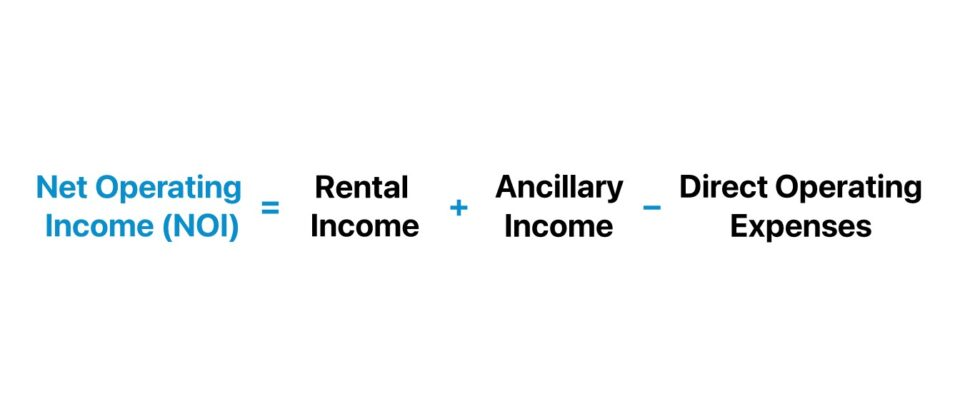• NOI stands for “Net Operating Income” and measures the profitability of an income-generating property prior to deducting financing costs and taxes.
• The net operating income (NOI) formula starts by calculating the sum of a property’s rental and ancillary income, which then subtracts any direct operating expenses incurred.
• NOI is intended to determine the profitability of a given property before accounting for the depreciation expense, financing costs (interest), income taxes, corporate-level SG&A expenses, tenant improvements, and capital expenditures (Capex).
• Net operating income (NOI) is part of the capitalization rate calculation, wherein NOI is divided by the market value of the property to arrive at the implied cap rate.

## How to Calculate Net Operating Income (NOI)?

NOI, stated in simple terms, reflects the income generated by a property upon subtracting direct operating expenses.

In practice, the net operating income (NOI) is a fundamental real estate metric because it is the standardized measure of profitability to analyze potential and existing property investments.

By determining the historical profitability of a property investment – which offers insights into the profit potential of the property on a pro forma basis – the net operating income (NOI) facilitates better-informed investing decisions.

NOI is not only a practical measure to analyze the profitability of a given property, but the metric is also well-suited for comparability among different property investment opportunities (i.e. closer to “apples-to-apples” comparisons) since the metric neglects financing costs, debt service, and capital expenditures (Capex).

## NOI Formula

The net operating income (NOI) formula is the sum of the property’s rental income and ancillary income, subtracted by its direct operating expenses.

Net Operating Income (NOI) = (Rental Income + Ancillary Income) Direct Operating Expenses

The NOI formula strives to isolate the core operating profits of real estate assets to avoid muddying the waters with non-operating items such as corporate overhead and non-cash items such as depreciation.

However, more important than what expenses factor into NOI are the expenses that do NOT impact NOI.

In particular, the NOI metric is intended to capture profitability before any depreciation, interest, income taxes, corporate-level SG&A expenses, capital expenditures (Capex), or financing costs.

Most real estate companies – such as real estate investment trusts (REITs) and real estate private equity (REPE) firms – own multiple properties in their portfolios, so accurately analyzing NOI requires isolating property-level profitability.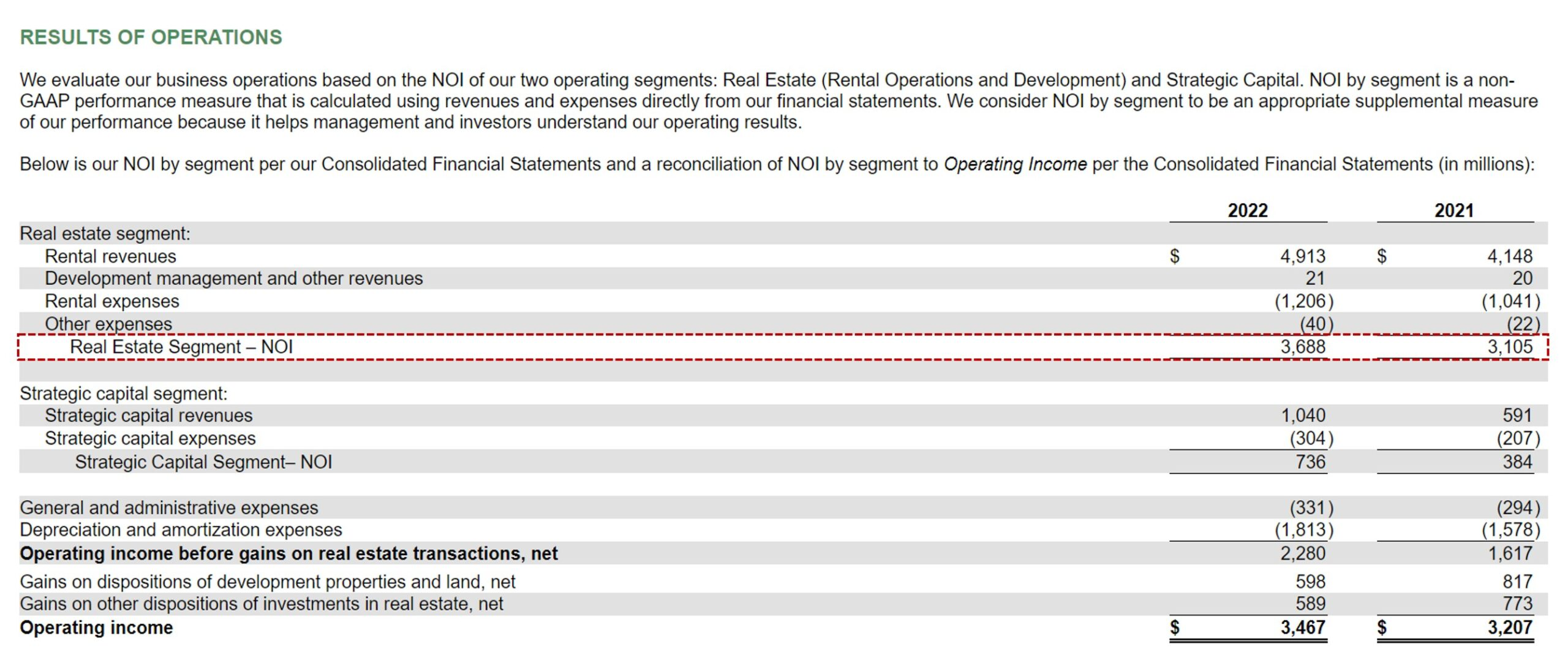Prologis REIT Real Estate Segment (RES) – NOI Example (Source: PLD 10-K)

## NOI vs. EBITDA: What is the Difference?

The net operating income (NOI) and earnings before interest, taxes, depreciation, and amortization (EBITDA) are both non-GAAP financial measures that are widely used in their respective industries.

• Net Operating Income (NOI) → The NOI metric is used in the real estate sector to measure a property’s potential profitability. The formula to compute NOI starts with calculating a property’s effective gross income (EGI) and subtracting the direct operating expenses, such as maintenance costs, repair fees, property management costs, utilities, and property taxes. Notably, the NOI metric neglects debt service payments, interest expense, capital expenditures (Capex), depreciation, and income taxes.
• EBITDA → The EBITDA metric is a widely-used measure of operating profitability used to analyze the operational performance of companies in all sorts of industries. EBITDA can be calculated as operating income (EBIT) plus depreciation and amortization (D&A) or net income plus interest, taxes, and D&A. Because EBITDA is independent of the capital structure decision (i.e. the percent mixture of debt and equity to fund operations), tax jurisdiction, and discretionary management decisions (e.g. the useful life assumption of fixed assets), the non-GAAP metric offers a clear picture into the operating performance of a company with regard to its operational efficiency and profitability.

## What is a Good NOI for a Rental Property?

The net operating income (NOI) of a rental property offers practical insights into the profitability of an investment, which carries broad implications on the implied valuation.

However, a “good” NOI is relatively subjective and contingent on several factors, including the property type, the location, and the current state of the real estate market.

Still, as a general rule of thumb, rental properties that generate positive NOI figures in excess of comparable properties are perceived positively in the market.

On the other hand, a property with a negative NOI signifies that the property is unprofitable, and thus it costs more to operate the property than the amount it earns in income on behalf of the investor.

To further determine the merits of a rental property using NOI, real estate investors often compare NOI to the market value of the property (or the purchase price, at times).

Comparing the NOI of a rental property to its market value at present yields the capitalization rate, or “cap rate”. Like NOI, the cap rate is a foundational metric used among real estate investors to analyze the viability of potential investments.

The formula to determine the cap rate is the ratio between net operating income (NOI) and the property value.

Cap Rate (%) = Net Operating Income (NOI) ÷ Property Value

By re-arranging the formula, the estimated property value can be derived.

Implied Property Value = Net Operating Income (NOI) ÷ Cap Rate (%)

For example, if the net operating income (NOI) of a property is \$4 million and its cap rate is 10%, the implied property value is \$40 million.

• Implied Property Value = \$4 million ÷ 10% = \$40 million

Generally speaking, properties with a cap rate of 4% to 12% are considered to be attractive investment opportunities, yet the surrounding details (e.g. location, property type) are all factors that ultimately determine the outcome.

Contrary to common misconception, a higher cap rate is not the priority to all real estate investors because, per usual, higher potential returns coincide with greater levels of risk.

Therefore, the target cap rate depends on the specific investment firm’s strategy with regard to their returns threshold and appetite for risk.

## What is a Good NOI Margin?

For comparability, the net operating income (NOI) must be standardized into a margin (to be “apples-to-apples”).

The NOI margin is a profitability ratio that measures the operating efficiency of a property and is calculated by dividing net operating income (NOI) by the total property revenue.

The resulting figure must be multiplied by 100 to express the margin as a percentage.

Conceptually, the NOI margin answers the question, “How much of each dollar earned in revenue is kept as net operating profits (NOI)?”

The higher the NOI margin, the greater the proportion of property revenue that is converted into net operating income (NOI).

• Higher NOI Margin (%) → Efficiently Operated Property
• Low NOI Margin (%) → Inefficiently Operated Property

But rather than analyzing the NOI margin on a standalone basis, the profit margin should be compared to similar properties in terms of property type, class, and location to have a relative benchmark to underpin the comparative analysis.

## NOI Calculator

We’ll now move on to a modeling exercise, which you can access by filling out the form below.#### Excel TemplateSubmitting...

## Net Operating Income Calculation Example (NOI)

Suppose we’re tasked with calculating the net operating income (NOI) of the real estate segment of Prologis (NYSE: PLD) in fiscal years 2021 and 2022 using the following data.

Select Financial Data – Real Estate Segment (RES)
(\$ in millions) 2021A 2022A
Rental Revenues \$4,148 \$4,913
Development Management and Other Revenues \$20 \$21
Rental Expenses (\$1,041) (\$1,206)
Other Expenses (\$22) (\$40)

The revenue component of the NOI formula is the sum of “Rental Revenues” and “Development Management and Other Revenues,” whereas the direct operating expenses component is the sum of “Rental Expenses” and “Other Expenses.”

For 2021 and 2022, the net operating income (NOI) of the real estate segment (RES) comes out to \$3,105 million and \$3,688 million, respectively.

• Net Operating Income (NOI), FY-2021 = \$4,148 million + \$20 million – \$1,041 million – \$22 million = \$3,105 million
• Net Operating Income (NOI), FY-2022 = \$4,913 million + \$21 million – \$1,206 million – \$40 million = \$3,688 million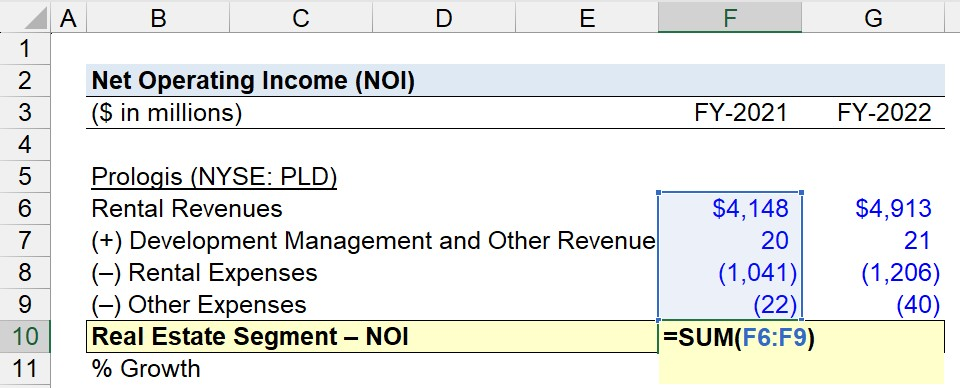In conclusion, the net operating income (NOI) of the real estate segment of Prologis grew by approximately \$583 million year-over-year (YoY) from 2021 to 2022, at an implied growth rate of 18.8%.

• Net Operating Income (NOI) – YoY Growth Rate = (\$3,688 million ÷ \$3,105 million) – 1
• NOI – YoY Growth Rate = 18.8%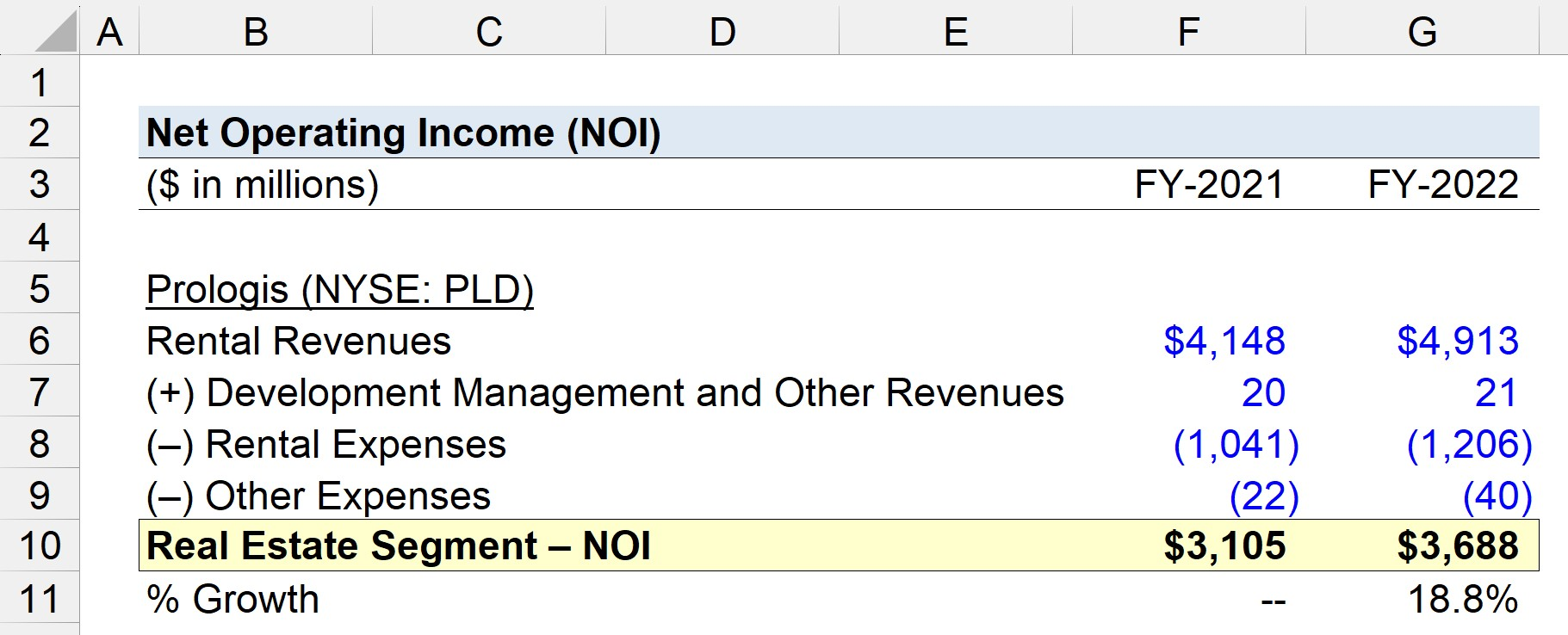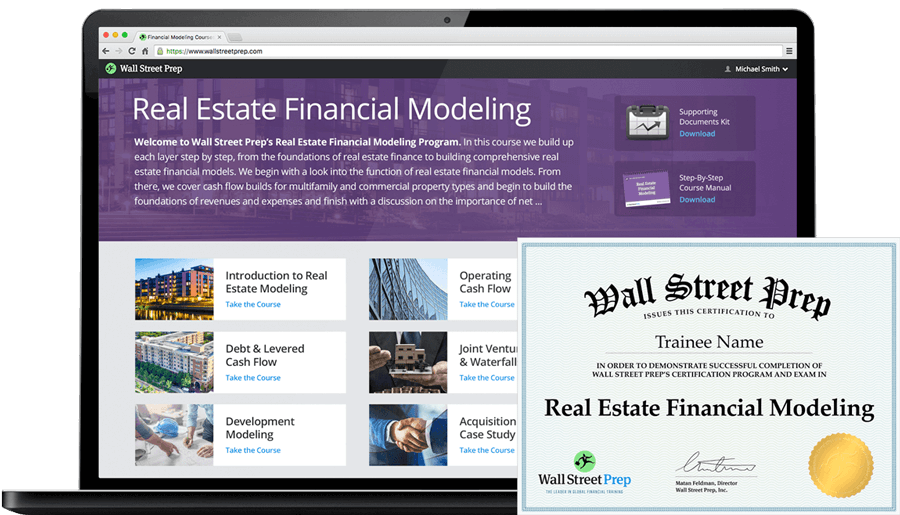20+ Hours of Online Video Training

### Master Real Estate Financial Modeling

This program breaks down everything you need to build and interpret real estate finance models. Used at the world's leading real estate private equity firms and academic institutions.